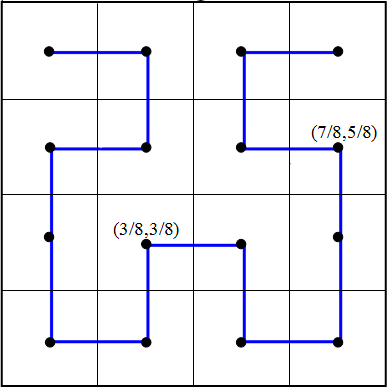Monday, February 10, 2014

Further Properties of Space-filling Curves

This is the final part of a three-part post. For the first, see here.

In the previous post, we examined an iterative sequence of curves, the limit of which after an infinite number of iterations is called the Hilbert curve. We now prove that the Hilbert curve indeed has the property it was constructed to have: it fills the unit square. Consider the first iteration again.The first iteration includes all four points of the form (m/2,n/2) when m and n are each either one or three. In binary decimal notation, 1/4 = 0.01 and 3/4 = 0.11. To see the relevance of this fact, now consider the second iteration.The points along the curve marked by black dots are of the form (m/8,n/8) with m and n both odd (and between 0 and 8), and two of these points are explicitly marked with their coordinates for clarity. It is obvious that the second iteration contains all points of this form. In binary notation, the points are of the form (0.ab1,0.cd1), where a, b, c, and d can stand for either 0 or 1. For example, (7/8,5/8) = (0.111,0.101). Since each iteration contains smaller copies of the previous one, it is clear that this pattern continues, and the third iteration will include all points of the form (m/16,n/16), with 0 < m, n < 16 and both m and n odd. In decimal notation, the curve includes all points each of whose coordinates has a 4-digit terminating binary expansion that ends in 1 (just as the second iteration had 3-digit terminating binary expansions ending in 1).

Next, we must note that any binary expansion can be approximated to arbitrary accuracy by others ending in the digit "1". For example, let P be the point in the unit square (0.101010101...,0.10100000...), where the repetition in the first expansion continues infinitely. Now consider the sequence {(0.11,0.11), (0.101,0.101),(0.1011, 0.1011),(0.10101,0.10001),...}. The nth point in this sequence lies on the curve of the nth iteration, and the sequence converges to the point P defined above. Thus the point P lies on the limit of the iterations, i.e., the Hilbert Curve itself. Note that the second coordinate of point P is exactly matched by the point on the 2nd iteration, and the sequence goes on to actually veer away (temporarily) from its final value. However, it still approaches the same limit as the sequence continues.

Since binary is simply another way of writing the real numbers, it is easy to show that there is a binary expansion for every real number from 0 to 1. For more discussion on this topic, see The Infinity Series, specifically here. Therefore, the Hilbert Curve visits every point in (and on) the unit square, and is indeed a space-filling curve. We note next that the Hilbert curve actually "overachieves" its goal; it actually visits several points of the unit square multiple times. For example, the point (1/2,1/2) is the limit of the sequence {(0.11,0.11), (0.101,0.101),(0.1001, 0.1001),(0.10001,0.10001),...} as well as of another sequence {(0.01,0.01), (0.011,0.011),(0.0111, 0.0111),(0.01111,0.01111),...}. Thus the function mapping the unit interval onto the Hilbert Curve is not one-to-one though it is continuous. Contrast this with the first function we constructed from the unit interval onto the unit square, which was one-to-one (after resolving the issue of multiple decimal representations) but not continuous.

It is impossible for a function from the unit interval to the unit square to be one-to-one and continuous. Such functions are called homeomorphisms, and two spaces for which is it possible to construct a homeomorphism mapping one onto the other are topologically equivalent in a certain sense. This means that they share certain intrinsic toplogical properties. For example, if a homeomorphism from a space that is connected (see image below) to another space exists, the latter space must also be connected.Examples of connected and disconnected spaces.    Connected spaces can only have one component (A),  while  disconnected ones have multiple disjoint components (B).

Also, two homeomorphic spaces (this means that there is a homeomorphism from one onto the other) share other connectedness properties. For example, the unit interval has the property that, when any point of it (except an endpoint) is removed, it becomes disconnected. However, the unit square cannot be disconnected by the removal of any single point. Hence the two spaces differ in a topological property that is conserved by homeomorphisms and are not homeomorphic. Therefore, a continuous space-filling curve that is also one-to-one is impossible.

It is also worth noting that the concept of a Hilbert curve can be extended to any finite dimension; in three dimensions, instead of filling the unit square, the curve fills the unit cube. This would be done by dividing the cube into 23 = 8 cubes of side length 1/2 and divding the unit interval into 8 corresponding subintervals for the first iteration, and so on. Generally, the Hilbert curve, like many other mathematical oddities, shatters our intuition when it comes to what properties mathematical objects can possess, and reminds us of the differences between the physical and mathematical worlds.

Sources: Counterexamples in Analysis by Bernard R. Gelbaum and John M. H. Olmsted, Space-filling Curve on Wikipedia, http://upload.wikimedia.org/wikipedia/commons/9/95/Connected_and_disconnected_spaces.svg